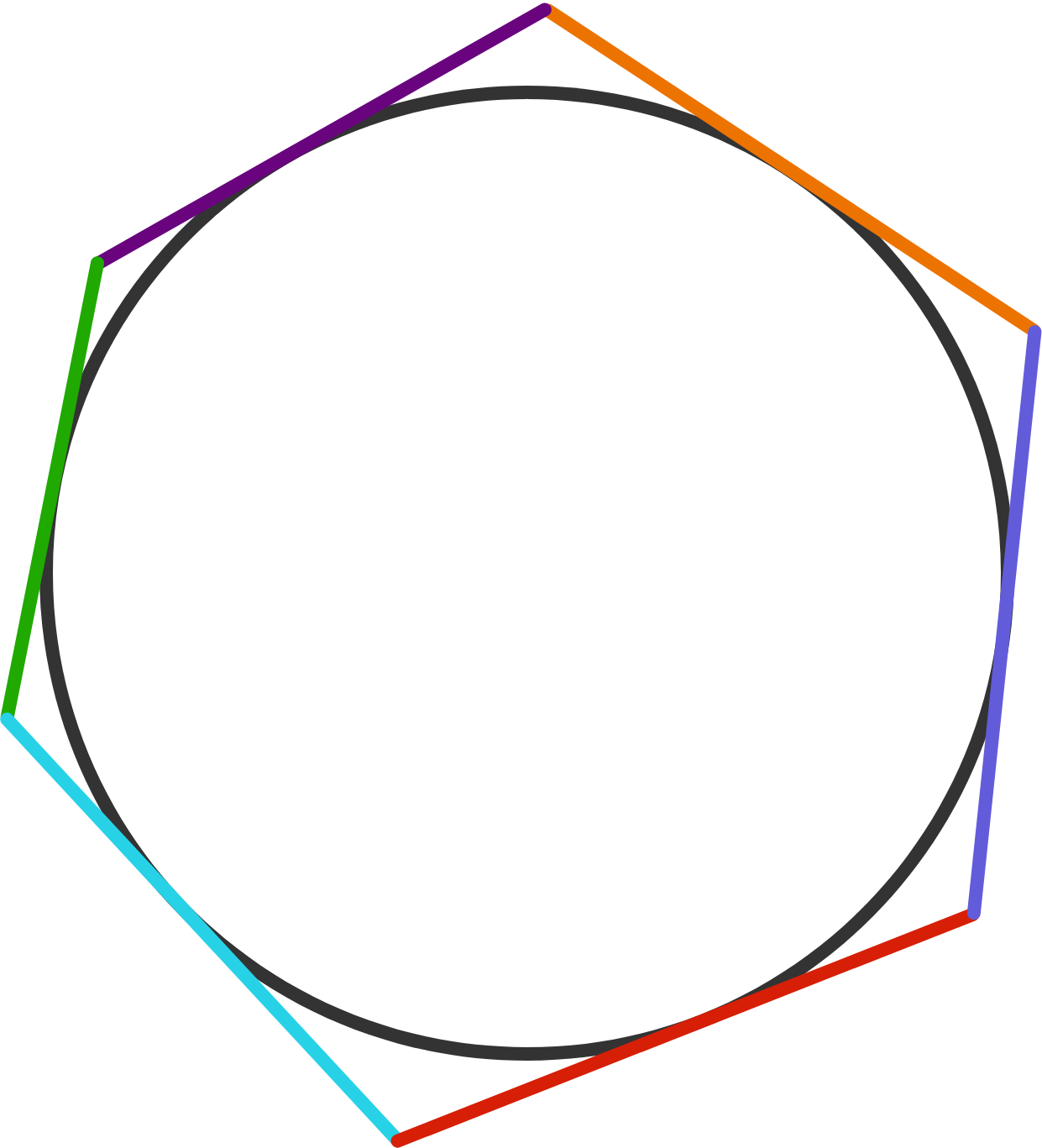# Problems of the Week

Contribute a problem

# 2017-07-24 IntermediateA kitten is inside each of two boxes, initially held at rest, as shown in the diagram. Kitty together with its box weighs $\SI[per-mode=symbol]{1}{\kilo\gram},$ and Petty together with its box weighs $\SI[per-mode=symbol]{1.5}{\kilo\gram}$.

When the boxes are released, which kitten will move down?

Notes

• Assume that the pulleys and strings are both massless and frictionless.

• The brown line at the bottom of the diagram shows that the other end of Kitty's rope is attached to the ground.

• When the boxes are released, they are simply let go. No ropes are cut!

The answer to the following question is an integer, which can also be expressed as $\frac{a}{b}$, where $a$ and $b$ are positive integers.

What is $2a^2-14ab+7?$

Hint: If you aren't familiar with self-referential puzzles, you can try this similar problem.A circle is inscribed in a hexagon, as shown in the diagram.

Is it possible that the side lengths of the hexagon are $7,9,11,13,15,17$ in some order?The dartboard in the diagram consists of an infinite number of concentric circles. Each successively smaller circle has $\frac{3}{4}$ the radius of the preceding, larger circle.

A dart is thrown somewhere on the dartboard (striking uniformly at random over the entire area of the dartboard).

To 2 decimal places, what is the probability it strikes black? Assume the point of the dart is one-dimensional.

\begin{aligned} a^2+b+c \\ b^2+c+a \\ c^2+a+b \end{aligned}

If $a, b, c$ are positive integers, then is it possible that all three of the numbers above are perfect squares?

×